# The Gyrate Rhombicosidodecahedron

The gyrate rhombicosidodecahedron is the 72nd Johnson solid, or J72 for short. It has 62 faces (12 pentagons, 30 squares, 20 triangles), 120 edges, and 60 vertices, just like the rhombicosidodecahedron, except that one of the pentagonal cupola (J5) segments has been gyrated so that the polyhedron is no longer vertex-transitive.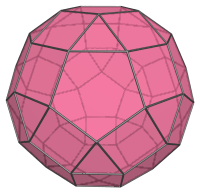J72 closely resembles the uniform rhombicosidodecahedron, but it can be recognized by noting the tell-tale pairs of adjacent squares and the triangles that share an edge with a pentagon: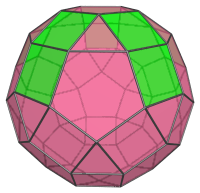The uniform rhombicosidodecahedron does not have square faces that share edges with each other, nor does it have triangles that share edges with pentagons. These features arise from the gyrated configuration of the pentagonal cupola segment highlighted below: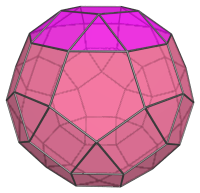## Projections

Here are some views of the gyrate rhombicosidodecahedron from various angles:

Projection Description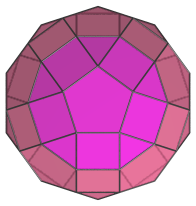Top view.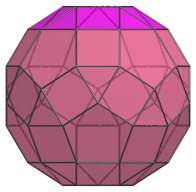Front view, parallel to pair of opposite triangles.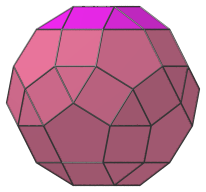Side view.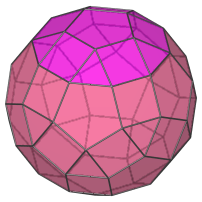Oblique view.

## Coordinates

The Cartesian coordinates of the gyrate rhombicosidodecahedron with edge length 2 are:

• (0, ±(φ+2), ±φ2)
• (±φ2, 0, −(φ+2))
• (−φ2, 0, (φ+2))
• (±(φ+2), ±φ2, 0)
• (±1, ±1, ±φ3)
• (±1, ±φ3, ±1)
• (±φ3, ±1, −1)
• (−φ3, ±1, 1)
• (±φ, ±φ2, ±2φ)
• (±φ2, ±2φ, ±φ)
• (±2φ, ±φ, −φ2)
• (−2φ, ±φ, φ2)
• ((8φ+1)/5, ±1, (6φ+7)/5)
• ((8φ+6)/5, ±φ, (φ+7)/5)
• ((13φ+1)/5, 0, (φ+2)/5)

where φ=(1+√5)/2 is the Golden Ratio.

Last updated 05 Apr 2019.